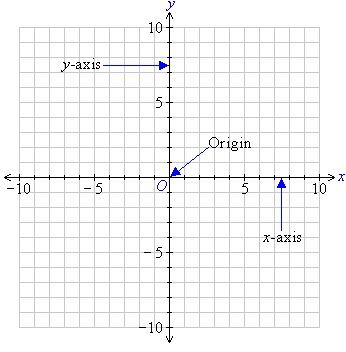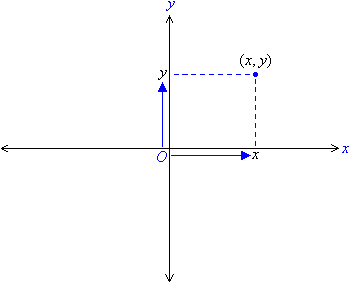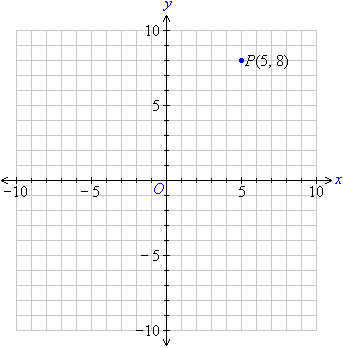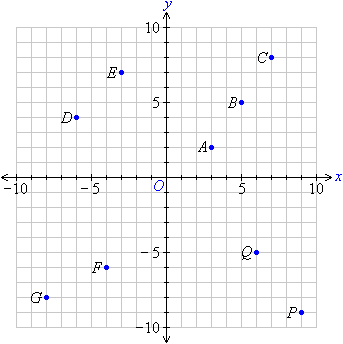Year 8 Interactive Maths - Second Edition

## The Cartesian Plane

The Cartesian plane consists of two directed lines that perpendicularly intersect their respective zero points.The horizontal directed line is called the x-axis and the vertical directed line is called the y-axis.  The point of intersection of the x-axis and the y-axis is called the origin and is denoted by the letter O.

### The Coordinates

The position of any point on the Cartesian plane is described by using two numbers:  (x, y).  The first number, x, is the horizontal position of the point from the origin.  It is called the x-coordinate. The second number, y, is the vertical position of the point from the origin.  It is called the y-coordinate.  Since a specific order is used to represent the coordinates, they are called ordered pairs.For example, the ordered pair (5, 8) represents a point 5 units to the right of the origin in the direction of the x-axis and 8 units above the origin in the direction of the y-axis as shown in the diagram below.

###### We say that:

The x-coordinate of point P is 5; and the y-coordinate of point P is 8.

###### Or simply, we can say that:

The coordinates of point P are (5, 8).###### Note the following:
• For the point P(5, 8), the ordered pair is (5, 8).  So:
5 is the x-coordinate, and
8 is the y-coordinate.
• P(5, 8) means P is 5 units to the right of and 8 units above the origin.

#### Example 1

State the coordinates of each of the points shown on the Cartesian plane:##### Solution:

A is 3 units to the right of and 2 units above the origin.  So, point A is (3, 2).
B
is 5 units to the right of and 5 units above the origin.  So, point B is (5, 5).
C
is 7 units to the right of and 8 units above the origin.  So, point C is (7, 8).
D
is 6 units to the left of and 4 units above the origin.  So, point D is (–6, 4).
E
is 3 units to the left of and 7 units above the origin.  So, point E is (–3, 7).
F
is 4 units to the left of and 6 units below the origin.  So, point F is (–4, –6).
G
is 8 units to the left of and 8 units below the origin.  So, point G is (–8, –8).
P
is 9 units to the right of and 9 units below the origin.  So, point P is (9, –9).
Q
is 6 units to the right of and 5 units below the origin.  So, point Q is (6, –5).

###### Key Terms

Copyright © 2000-2019 mathsteacher.com Pty Ltd.  All rights reserved.
Australian Business Number 53 056 217 611

Copyright instructions for educational institutions

Please read the Terms and Conditions of Use of this Website and our Privacy and Other Policies.
If you experience difficulties when using this Website, tell us through the feedback form or by phoning the contact telephone number.